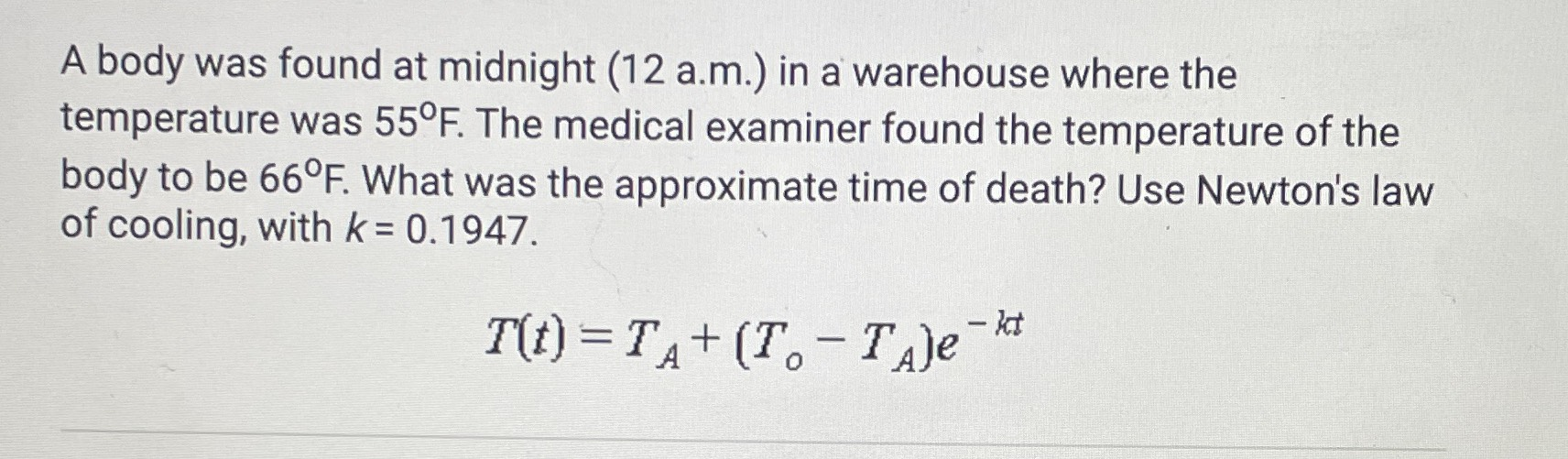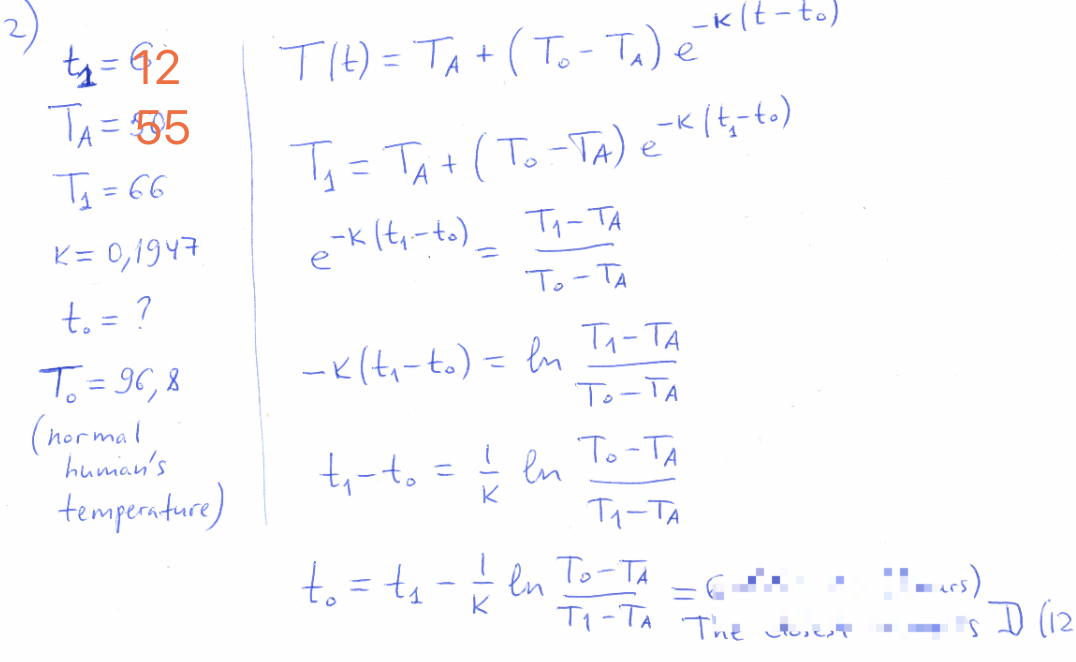### ¿Todavía tienes preguntas de matemáticas?

Pregunte a nuestros tutores expertos
Algebra
PreguntaA body was found at midnight (12 a.m.) in a warehouse where the temperature was $$55 ^ { \circ }$$ F. The medical examiner found the temperature of the body to be $$66 ^ { \circ } F$$ . What was the approximate time of death? Use Newton's law of cooling, with $$k = 0.1947$$ .

$$T ( t ) = T _ { A } + ( T _ { o } - T _ { A } ) e ^ { - k t }$$